All submissions of the EM system will be redirected to Online Manuscript Submission System. Authors are requested to submit articles directly to Online Manuscript Submission System of respective journal.

# Cylindrical Shock Waves in a Low Conducting Gas in Presence of Axial and Azimuthal Components of Magnetic Induction

Department of Mathematics and Statistics, D.D.U. Gorakhpur University, Gorakhpur-273009, India.

*Corresponding Author:
Department of Mathematics and Statistics
D.D.U. Gorakhpur University
Gorakhpur-273009, India.

Received date: 16/09/2013 Revised date: 20/09/2013 Accepted date: 28/09/2013

Visit for more related articles at Research & Reviews: Journal of Pure and Applied Physics

## Abstract

The propagation of diverging cylindrical shock waves in a low conducting gas under the influence of spatially variable axial and azimuthal components of magnetic induction, by using the method of self-similarity. The initial density of the medium is assumed either to be uniform or to obey a power law. Also, both the components of the initial magnetic induction are taken to vary as some power of the distance from the axis of symmetry. The total energy of the flow-field behind the shock is constant or increasing due to instantaneous or time dependent energy input. The effects of variation of the initial density and the ratio of specific heats of the gas, on the propagation of shock and the flow-field behind it are investigated.

#### Keywords and Phrases

Shock waves, self-similar flow, variable initial density, variable initial magnetic induction, variable energy input, low electrical conductivity.

#### Introduction

Lin  has extended the Taylor’s analysis  of the intense spherical explosion to the cylindrical case. The law of variation of the radius of a strong cylindrical shock wave produced by a sudden release of a finite amount of energy was obtained. Applying the results of this analysis to the case of hypersonic flight, it was shown that the shock envelope behind a meteor or a high speed missile is approximately a paraboloid.

Since at high temperatures that prevail in the problems associated with shock waves a gas is ionized, electromagnetic effects may also be significant. A complete analysis of such a problem should therefore consist of the study of the gasdynamic flow and the electromagnetic field simultaneously. The study of the propagation of cylindrical shock waves in a conducting gas in presence of an axial or azimuthal magnetic induction is relevant to the experiments on pinch effect, exploding wires and so forth. This problem both in the uniform or non-uniform ideal gas was undertaken by many investigators, for example, Pai , Cole and Greifinger , Sakurai , Bhutani , Christer and Helliwell , Deb Ray , Vishwakarma and Yadav . One of the basis assumptions of these works is that the shock wave is propagated in a gaseous medium as a result of an instantaneous release of energy along a line.

While the assumption of instantaneous energy input is considered adequate for most problems, there are processes in which the energy input, though very rapid, can be considered to be time dependent. Examples of time dependent energy input are the arc discharges, exploding wire phenomena and chemical energy release (as might occur in two phase detonations). Freeman  has considered the propagation of shock waves resulting from variable energy input. He has paid special attention to the case of cylindrical symmetry in view of its particular application to the problem of cylindrical spark channel formation from exploding wires (Freeman and Craggs ).

In the present work, we studied the propagation of diverging cylindrical shock waves in a low conducting and uniform or non-uniform gas as a result of time dependent energy input, under the influence of spatially variable axial and azimuthal components of magnetic induction. The medium ahead and behind the shock front are assumed to be an inviscid one and to behave as a thermally perfect gas. The initial density of the gas is assumed to be uniform or to vary as some power of distance. The total energy of the flow-field behind the shock is not constant, but increasing due to time dependent energy input. The gas ahead of the shock is assumed to be at rest. Effects of viscosity, heat-conduction, radiation and gravitation are not taken into account. Distribution of the flow variables between the shock front and the inner expanding surface are obtained, and the effects of the variation of initial density and the ratio of specific heats are investigated.

Fundamental Equations and Boundary Conditions

The basic equations governing the unsteady and cylindrically symmetric motion of a low conducting gas in presence of axial and azimuthal components of magnetic induction are given by (Tyl , Sakurai  and Vishwakarma )whereare the density, velocity, pressure, axial magnetic induction and azimuthal magnetic induction, respectively, at distance r from the axis of symmetry and at the time t,is the initial axial magnetic induction,the initial azimuthal magnetic induction, γ the ratio of specific heats, μ the magnetic permeability, σ the electrical conductivity and ‘a’ the speed of sound given byThe internal energy per unit mass of the gas e is given byIt is assumed that, due to explosion along the axis of symmetry, a cylindrical shock is produced and propagates into the low conducting gas of density Ã¯ÂÂ²0 in presence of the axial magnetic inductionand azimuthal magnetic inductionThe density of the gas and the axial and azimuthal components of magnetic induction ahead of the shock are assumed to be varying and obeying the laws:where R is the shock radius and A, α, S, Q, m, n are constants.

Since σ is small, the magnetic induction may be taken continuous across the shock front (Sakurai ). Neglecting the counter pressure, the shock conditions may be written aswhere the subscript s refers the conditions immediately behind the shock front anddenotes the velocity of the shock.

#### Similarity Transformations

To obtain similarity solutions, we write the unknown variables in the following form (Vishwakarma and Yadav )where f, D, P, bz, and b are the functions of the non-dimensional variableonly. The shock front is represented by x = 1.

The total energy of the flow-field behind the shock is not constant, but assumed to be time dependent and varying as (Rogers , Freeman , Director and Dabora ).(3.2)

where E0 and k are constants. The positive values of k corresponds to the class in which the total energy increases with time. Since the flow is adiabatic and the shock is strong, this increase can only be achieved by the pressure exerted on the fluid by an expanding surface (a contact surface or a piston). The situation very much of the same kind may prevail in the formation of cylindrical spark channel from exploding wires. In addition, in usual cases of spark breakdown, time dependent energy input is a more realistic assumption than instantaneous energy input (Freeman ).

The total energy of the flow between the shock front and the inner expanding surface (piston) is therefore expressed as(3.3)

where rp is the radius of the inner surface.

Applying the similarity transformations (3.1) in the relation (3.3), we find that the motion of the shock front is given by the equation(3.4)

wherexpbeing the value of the x at the inner expanding surface.

Equation (3.4), on integration, yieldsand therefore,After using the similarity transformations, the equations (2.1) to (2.5) change into the following set of ordinary differential equations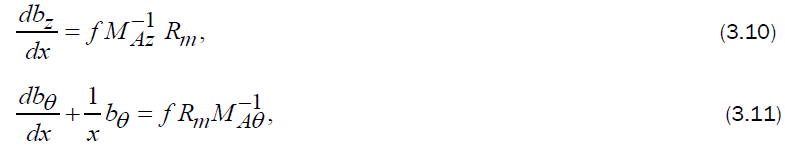whereare respectively, the magnetic Reynolds number, axial Alfven-Mach number and azimuthal Alfven-Mach number, and they are given byUsing the self-similarity transformations (3.1), the boundary conditions (2.10) can be written asFor the existance of similarity solutions magnetic Reynolds number Rm, axial Alfven-Mach number MAz and azimuthal Alfven-Mach number MAÃ¯ÂÂ± should be constants. Therefore,whereBy solving equations (3.7) to (3.9) for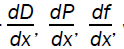we get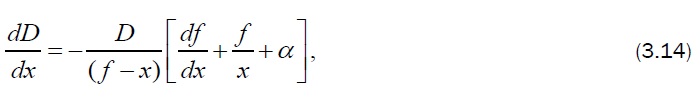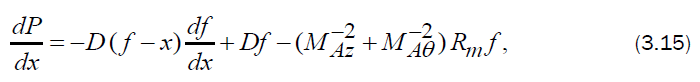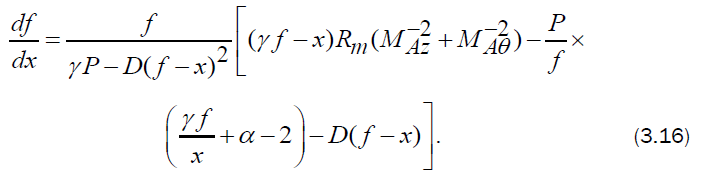The condition to be satisfied at the inner expanding surface is that the velocity of the fluid is equal to the velocity of the surface itself. This kinematic condition, can be written asFor exhibiting the numerical solutions it is convenient to write field variables in the following form:The shock-boundary conditions in terms of these variables areNow, the differential equations (3.10), (3.11) and (3.14) to (3.16) may be numerically integrated, with the boundary conditions (3.12) to obtain the flow-field between the shock front and the inner expanding surface.

#### Results and Discussions

The reduced flow variables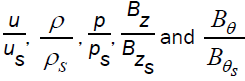are obtained by numerical integration of the differential equations (3.10), (3.11) and (3.14) to (3.16) with the boundary conditions (3.12). For the purpose of numerical integration, the values of the constant parameters are taken as (Vishwakarma and Singh ) γ = 1.4,1.66;The value α = 0 corresponds to the case of shock wave generated by instantaneous energy release (k = 0) in a gas of uniform density.

Figures 1-5 show the variation of the flow variableswith x at various values of the parameters α and γ. It is shown that, as we move inward from the shock front towards the inner expanding surface, the reduced fluid velocitydecreases for lower values of α (= 0,0.5), but it increases for comparatively higher values of α (= 1.5, 2.0); and the reduced densityreduced pressureand reduced axial magnetic inductiondecrease for all acceptable values of α, whereas reduced azimuthal magnetic inductionincreases. Table 1 shows the dimensionless position of the inner expanding surface xp at different values of α and γ.

The effects of an increase in the density variation index α are (from figures 1-5):

(i) to increase the velocityand the pressureat any point in the flow-field behind the shock;

(ii) to decrease the densityand the axial magnetic induction(iii) to decrease the slope of profiles of velocity and pressure and to increase that of the profiles of the density and axial magnetic induction; and

(iv) to decrease the distance (1−xp) of the inner expanding surface from the shock front (see table 1). This means that the ratio of the velocity of inner expanding surface to that of the shock front increases which results in more compression of gas behind the shock and an increases in the shock strength.

In fact, by an increase in α, k is increased which increases the time dependent energy input (through equation (3.2)) causing an increase in the velocity of inner expanding surface and hence an increase in the shock strength. The effects of an increase in the γ are (from figures 1-5):

(i) to decrease the velocityand to increase the densityand the axial magnetic inductionat any point in the flow-field behind the shock;

(ii) to decrease the slope of profiles of density and axial magnetic induction; and

(iii) to increase slightly the distance of inner expanding surface to the shock front (table 1).

Variation in α or γ has no effect on the azimuthal magnetic fieldin the flow-field behind the shock.

Equations (3.5) and (3.13) show that the shock velocity ÃÂR decreases with time and varies as t−1/2.

Table 1. Position of the inner expanding surface xp at different values of α and γ for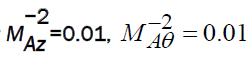and Rm=0.01.

#### Conclusions

In the present paper, the similarity solutions have been obtained for the propagation of cylindrical shock waves in a low conducting gas under the influence of variable initial axial and azimuthal components of magnetic induction. On the basis of the present study, one may draw the following conclusions:

(i) The shock velocity decreases with time as t−1/2.

(ii) An increase in the value of density variation index α gives rise an increase in the velocityand in the pressure, and a decrease in the densityand in the axial magnetic inductionat any point in the flow-field behind the shock.

(iii) An increase in α, increases the time dependent energy input, and hence increases the shock strength.

iv) An increase in the value of γ gives rise an increase in the densityand in the axial magnetic induction,and a decrease in the velocityat any point in the flow-field behind the shock.

#### References